# 业务驱动的电力通信网告警–故障关联分析Service Driven Alarm-Fault Correlation Analysis for Power Communication Network

• 全文下载: PDF(2979KB)    PP.1923-1935   DOI: 10.12677/CSA.2018.812214
• 下载量: 386  浏览量: 1,560   国家科技经费支持

At present, the power communication network transmission network carries a lot of key services. Therefore, network security and stable operation are to protect the basis of power security. In order to make the power communication network from the fault after the passive response into active defense, there needs an effective fault impact analysis scheme based on service-driven. However, the current research is mainly related to network fault alarms, lacking theoretical support and practical tools for service view. Therefore, in order to implement the modeling of the network fault impact on the services, this paper proposes a service-driven power communication network alarm-fault correlation analysis. First, from the services perspective, we build a bottom-up interaction relationship among different layers of the network. After that, we build a services risk correlation model to map the impact of the underlying network fault roots to different alarm levels. And then a mapping model between alarms and service risks is proposed. Finally, based on the above simulation architecture, a set of simulations system is achieved to verify the effectiveness of the simulation architecture.

1. 引言

2. 电力通信网诱因–故障告警关联分析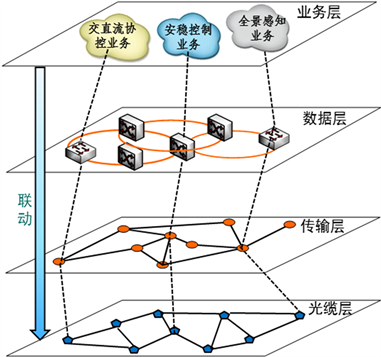Figure 1. Hierarchical topology of power communication network

2.1. 电力通信业务承载交互模型分析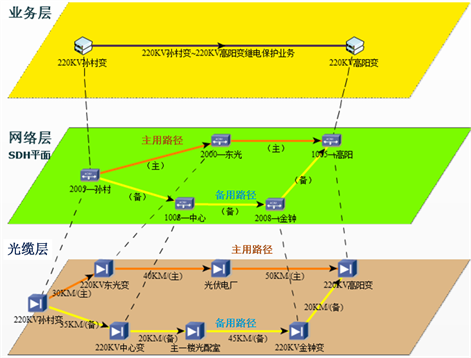Figure 2. Three-layer service model of power communication network

2.2. 业务驱动的故障关联分析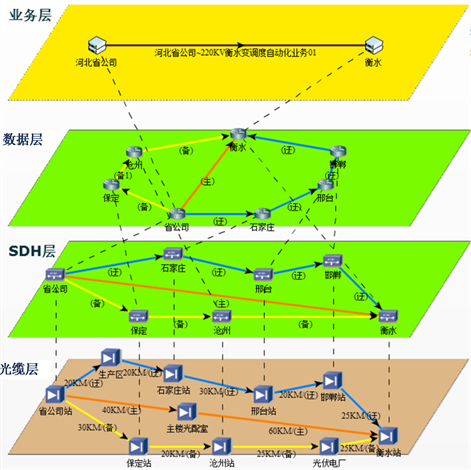Figure 3. Power communication network four-layer service model

2.3. 电力通信网诱因与告警之间的关联性建模

${\sum }_{{F}_{k}\in F}|{F}_{k}|=M$ (1)

${P}_{j}^{\text{G}}\left(t\right)=\text{1}-\underset{{F}_{k}\in {F}_{G}}{\prod }\left(1-{P}_{kj}^{\text{F}}\left(t\right)\right)$ (2)

$\begin{array}{l}{P}_{kj}^{\text{F}}\left(t\right)={\sum }_{i\in {F}_{k}}{w}_{i}{x}_{ij}{P}_{i}\left(t\right)-{\sum }_{i (3)

$\text{min}{\text{Z}}_{\text{1}}\left(W\right)={P}_{kj}^{\text{F}}\left(t\right)$ (4)

$\forall {F}_{k},{\sum }_{i\in {F}_{k}}{w}_{i}{x}_{ij}\frac{\partial {P}_{kj}^{\text{F}}\left(t\right)}{\partial {P}_{i}\left(t\right)}=1$ (5)

$\mathrm{min}{\text{Z}}_{\text{2}}\left(W\right)=-{\sum }_{i\in {F}_{k}}{w}_{i}{x}_{ij}\frac{\partial {P}_{kj}^{\text{F}}\left(t\right)}{\partial {P}_{i}\left(t\right)}{\mathrm{log}}_{2}\left({w}_{i}{x}_{ij}\frac{\partial {P}_{kj}^{\text{F}}\left(t\right)}{\partial {P}_{i}\left(t\right)}\right)$ (6)

$\begin{array}{l}\mathrm{min}\text{Z}\left(W\right)=\eta {\text{Z}}_{\text{1}}\left(W\right)+\left(\text{1}-\eta \right){\text{Z}}_{\text{2}}\left(W\right)\\ s.t.\left\{\begin{array}{l}{\sum }_{i\in {F}_{k}}{w}_{i}{x}_{ij}\frac{\partial {P}_{kj}^{\text{F}}\left(t\right)}{\partial {P}_{i}\left(t\right)}=1\\ \text{0}<{w}_{i}<1\end{array}\end{array}$ (7)

2.3.1. 电力通信网故障与诱因关联优化模型简化

$\text{min}{\text{Z}}_{\text{1}}\left(W\right)={P}_{kj}^{\text{F}}\left(t\right)\doteq {\sum }_{i\in {F}_{k}}{w}_{i}{x}_{ij}{P}_{i}\left(t\right)$ (8)

$\frac{\partial {P}_{kj}^{\text{F}}\left(t\right)}{\partial {P}_{i}\left(t\right)}\doteq \frac{\partial {\sum }_{i\in {F}_{k}}{w}_{i}{x}_{ij}{P}_{i}\left(t\right)}{\partial {P}_{i}\left(t\right)}={w}_{i}$ (9)

$\begin{array}{l}\mathrm{min}\text{Z}\left(W\right)=-\eta {\sum }_{i\in {F}_{k}}{x}_{ij}{w}_{i}^{2}{\mathrm{log}}_{2}\left({x}_{ij}{w}_{i}^{2}\right)+\left(1-\eta \right)\left({\sum }_{i\in {F}_{k}}{w}_{i}{x}_{ij}{P}_{i}\left(t\right)\right)\\ s.t.\left\{\begin{array}{l}{\sum }_{i\in {F}_{k}}{x}_{ij}{w}_{i}^{2}=1\\ \text{0}<{w}_{i}<1\end{array}\end{array}$ (10)

2.3.2. 扩展的牛顿迭代法

2.3.3. 实例分析

² 自然因素(F1)：地震，强风，老鼠啃咬，火灾；

² 人为因素(F2)：挪动光纤，施工挖断；

² 设备因素(F3)：自然老化。

1) 首先，对自然因素的诱因概率进行建模，设地震级别为1~10级，强风分为1~12级，老鼠啃咬程度为1~4级，火灾分为1~5级。设地震的起始影响等级为4级，强风为6级，老鼠啃咬为2级，火灾为1级，此时正遭遇8级台风和1级老鼠啃咬危险。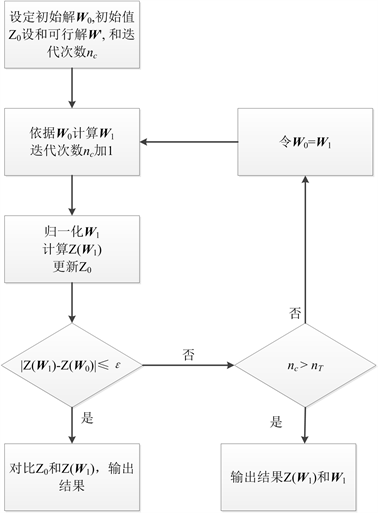Figure 4. Extended Newton iterative process flow chart

2) 对于人为因素，设挪动光纤的级别只有1，施工挖断的级别为2，两种因素的到达率分别为0.001和0.002，当前挪动光纤存在，且施工存在1级挖断影响可能性，因此则可以得到施工因素的发生概率分别为1−e −0.002和0.5 (1−e−0.01)。

3) 对于设备因素，设其使用时间为3年，使用寿命为5年，对应的发生故障概率为1−0.4e−3

$\mathrm{min}Ζ\left(W\right)=-\left({w}_{\text{2}}^{2}{\mathrm{log}}_{2}{w}_{\text{2}}^{\text{2}}+{w}_{3}^{2}{\mathrm{log}}_{2}{w}_{3}^{\text{2}}\right)+0.5\left(0.\text{33}{w}_{2}{\text{e}}^{-\text{2}}+0.\text{33}{w}_{3}{\text{e}}^{-\text{4}}\right)$

3. 业务风险模型构建

3.1. 业务状态分析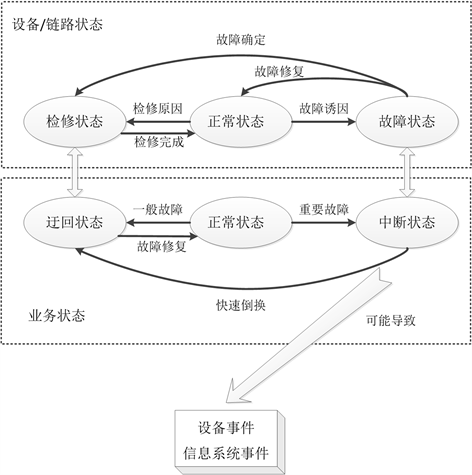3.2. 故障告警影响级别模型

${E}_{i}={P}_{i}\cdot {D}_{i}$ (11)

${D}_{i}={w}_{i}×{v}_{ih}×{C}_{i}$ (12)

vih为业务i所属种类h的权重。wi为业务si承载的设备和链路的权重和。Ci为业务权重，可参考文献  。

 (13)

4. 基于仿真的告警–故障关联风险分析实例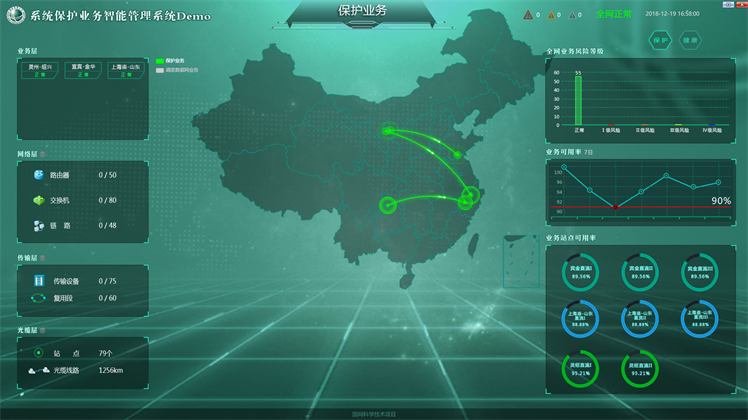Figure 6. General schematic diagram of the system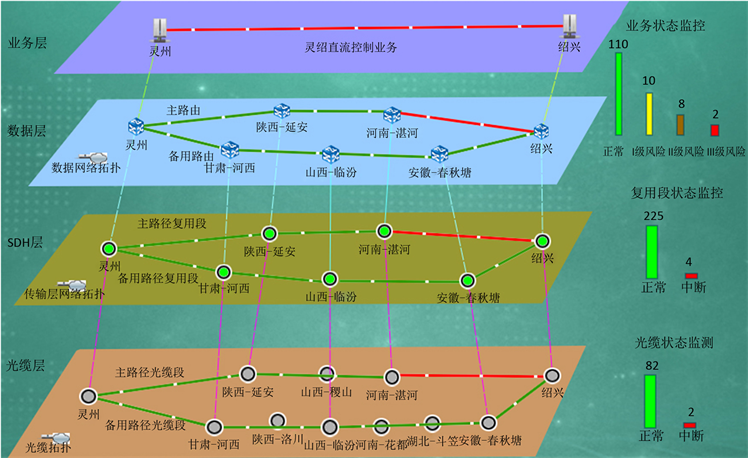Figure 7. Association topology diagram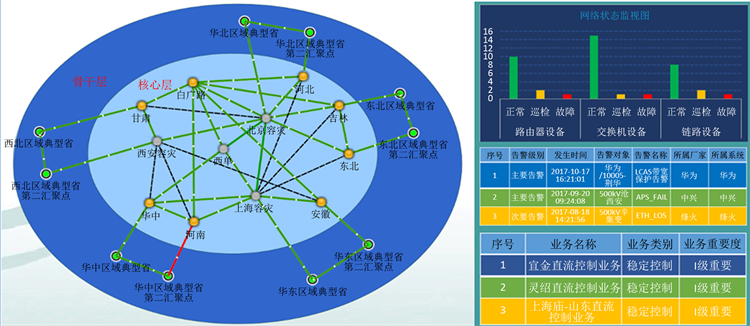Figure 8. Alarm display diagram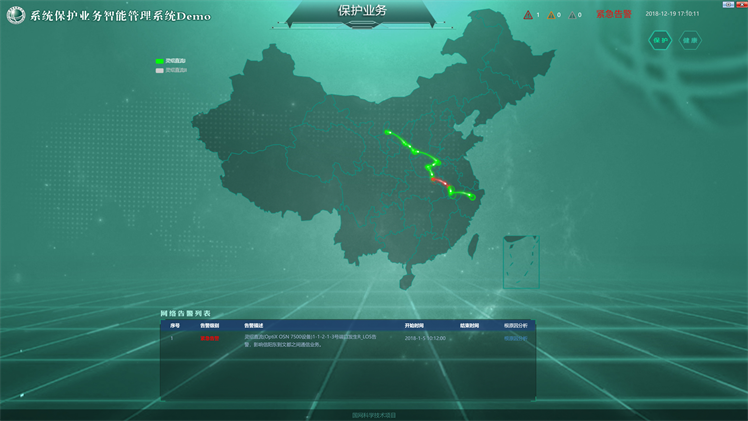Figure 9. Alarm cause analysis diagram

5. 结语

2017年国家电网公司总部科技项目：系统保护通信网络技术体制及关键技术研究(Grant No. SGXT0000ASJS1700054)。

  刘丽榕, 王玉东, 马睿, 孙雨潇. 电网系统保护业务分析及通信承载方案研究[J]. 电力信息与通信技术, 2017, 15(12): 12-18.  吴简, 李兴明. 基于关联规则的分布式通信网告警相关性研究[J]. 计算机科学, 2009, 36(11): 204-212.  谢万福, 李兴明. 基于模糊关联规则挖掘的多层网络告警相关性分析[D]: [硕士学位论文]. 成都: 电子科技大学, 2015.  李英敏, 高会生. 基于分层属性相似度的通信告警关联模型研究[D]: [硕士学位论文]. 北京: 华北电力大学, 2014.  赵振东, 黄楠, 李紫涵. SDH系统网络故障的告警关联分析[J]. 电力系统通信, 2012, 33(6): 63-66.  王腾. 电力系统通信网中SDH告警关联规则及故障诊断的研究[D]: [硕士学位论文]. 北京: 华北电力大学, 2014.  邓歆. 光传送网告警相关性分析及其应用的研究[D]: [博士学位论文]. 北京: 北京邮电大学, 2008.  邢宁哲, 卜亚杰, 常海娇, 喻鹏. 电力通信网安全事件量化模型和预测方法[J]. 电力信息与通信技术, 2016, 14(10): 120-126.  邓伟锋, 何文栋, 王明聚, 徐常志. 电力特种光缆断点快速修复技术研究及应用[J]. 工业技术创新, 2015(6): 597-602.  蒋康明, 曾瑛, 邓博仁, 唐瑞良. 基于业务的电力通信网风险评价方法[J]. 电力系统保护与控制, 2013(24): 101-106.